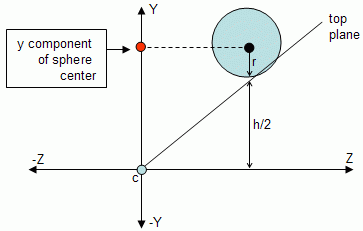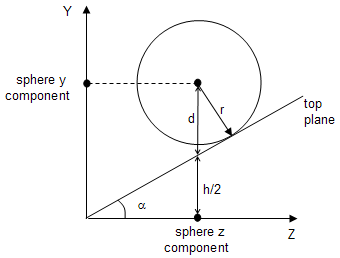Prev: Implementation Next: Implementation II

Under the radar approach testing spheres is far more complex than with the other methods, but nonetheless still more efficient.

Let’s start with the Z component. A sphere has a center c and a radius r. The Z component is the easiest of them all, and it must be performed first since it is required to know the z value of the sphere center in the camera referential.

First it is necessary to compute the z component of the sphere center in camera coordinates and this is performed as shown before.

```	v = p - cc
pc.z = v . Z```

The only difference in Z testing is the inclusion of the radius in the test condition. If pc.z is not between nearDist-r and farDist+r then p is outside the frustum.

```	if (pc.z > farDist +r || pc.z < nearDist - r)
return (OUTSIDE);```

Testing for interception can be achieved afterwards with the following code:

```	if (pc.z > farDist - r || pc.z < nearDist + r)
result = INTERSECT;```

Note that when the sphere is outside (red sphere) any of the given planes the testing can terminate, hence the return statement. However an intersection (orange sphere) with the near or far planes is inconclusive without testing the other coordinates first, therefore only the variable result is set in this case.Testing both x and y components is the delicate part. When both the x and y components are inside the frustum (the figure below shows only the Y axis) then the sphere is at least intersecting the frustum.But when is it totally inside, or outside? Consider the following figure where only the top plane is depicted to make the diagram simpler.If the only difference comparing to testing points would be the inclusion of the radius, then the sphere in the figure above would be outside when it clearly intersects the frustum. So the following code is not accurate:

```	if (pc.z > h/2 + r || pc.z < - h/2 - r)
return (OUTSIDE);```

The following figure shows that the distance we're looking for is not the radius r of the sphere but d.Fortunately d can be computed as a function of the radius and half the horizontal field of view angle (alpha in the figure). The angle between the vectors with the directions r and d is equal to alpha, hence:

`	d = r / cos(alpha)`

Since alpha is know a priori, the term 1/cos(alpha) can be precomputed so the extra computational effort during testing is only a multiplication by the radius. This term, hereafter named the sphereFactorY, needs to be computed when the perspective is set. A similar term for the X axis, sphereFactorX, is also required since the angle is potentially different (unless the ratio between width and height of the viewport is 1). The next section shows the implementation details.

 Prev: Implementation Next: Implementation II

### One Response to “Radar Approach – Testing Spheres”

1.I Think circle center “c” is actually referred to as p in the code 🙂

This site uses Akismet to reduce spam. Learn how your comment data is processed.